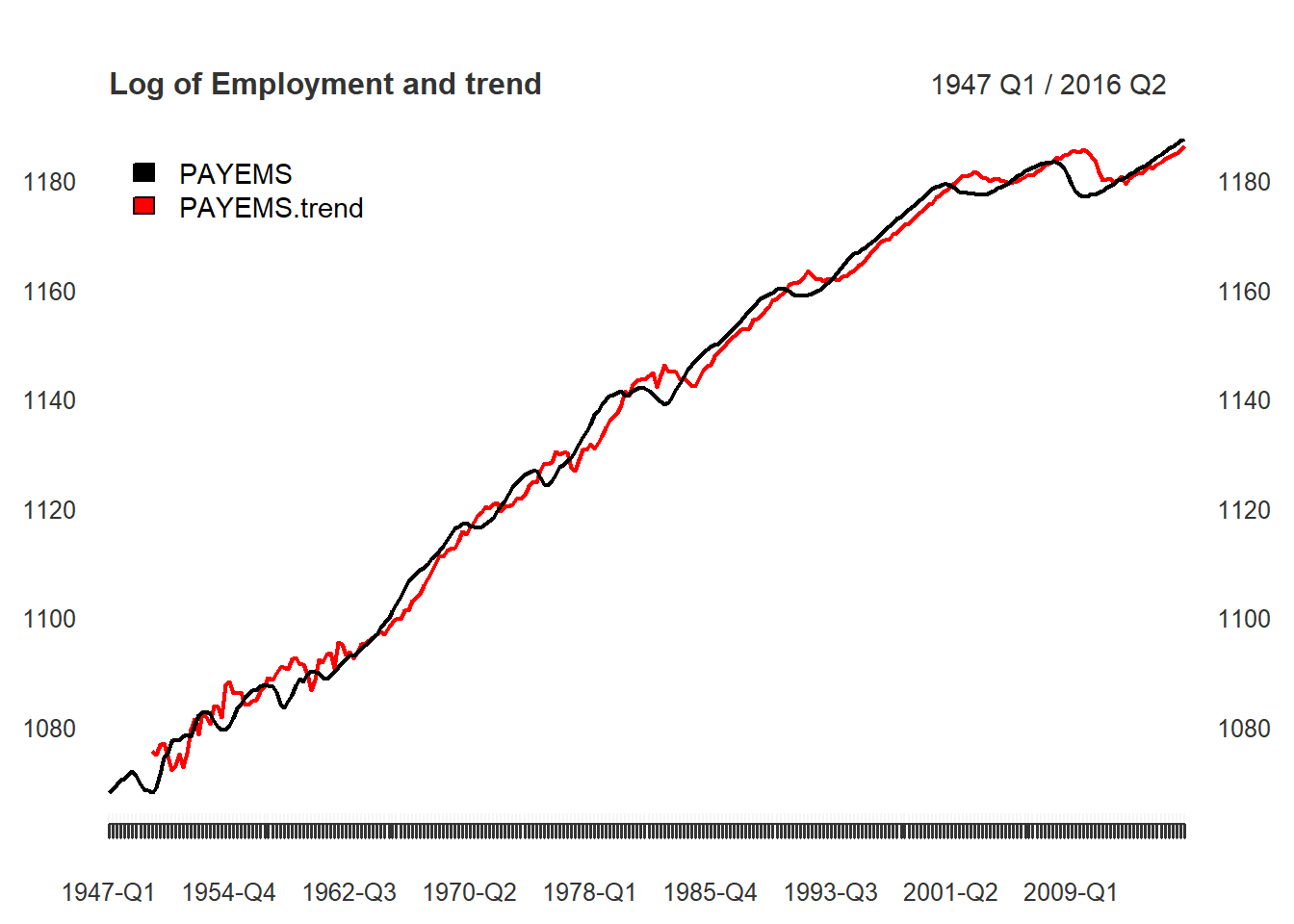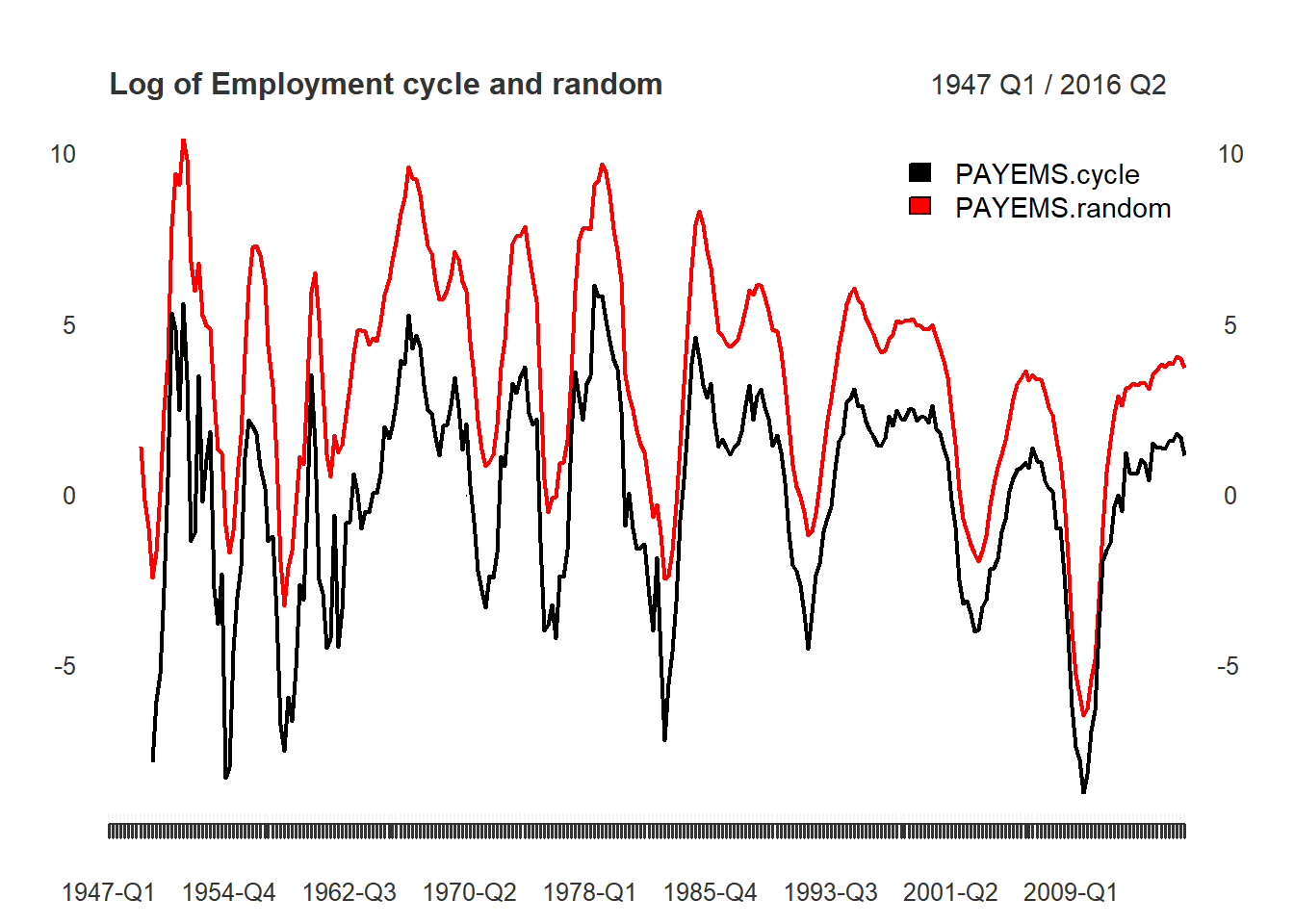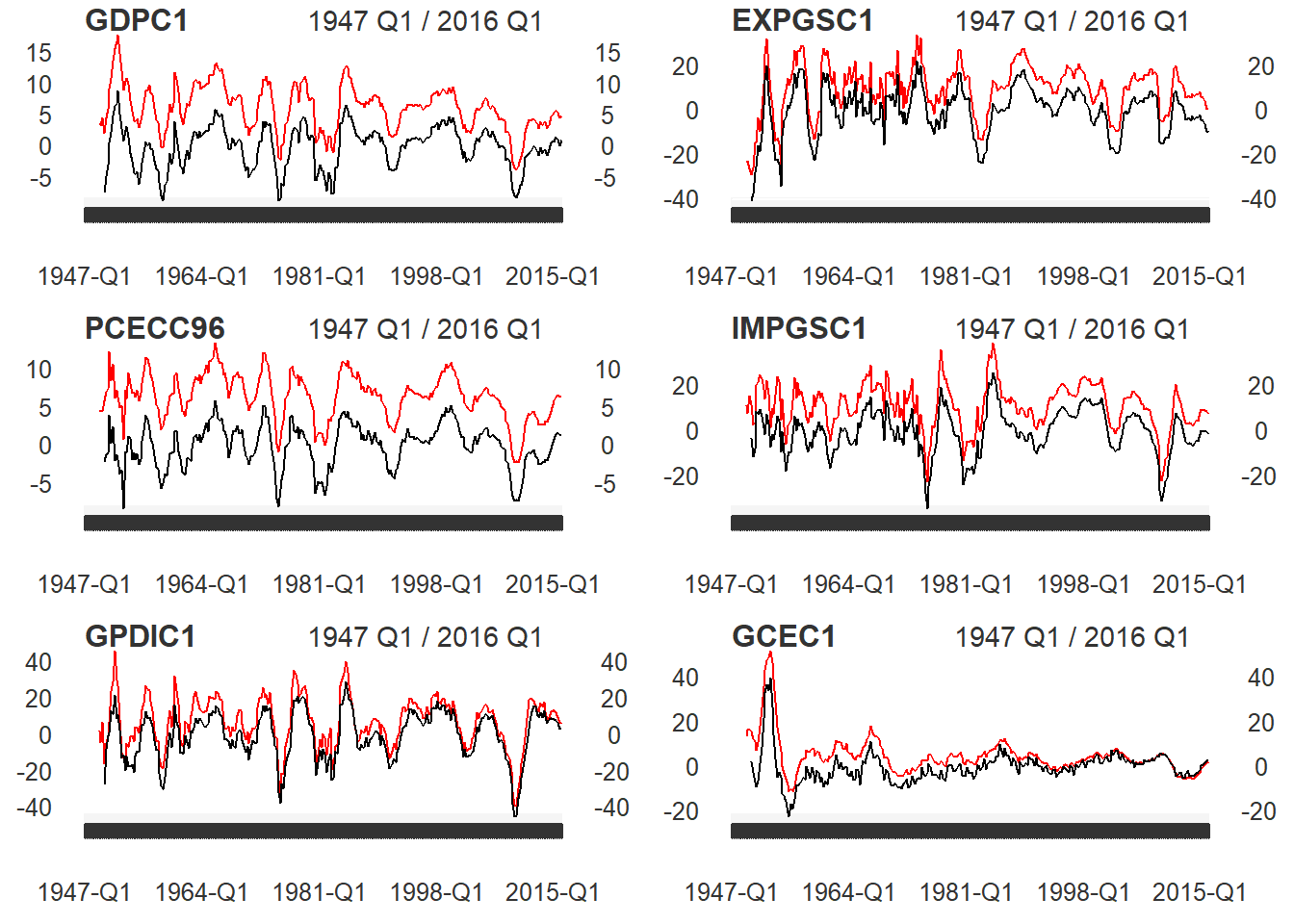In the working paper titled “Why You Should Never Use the Hodrick-Prescott Filter”, James D. Hamilton proposes an interesting new alternative to economic time series filtering. The neverhpfilter package provides functions for implementing his solution. Hamilton (2017) <doi:10.3386/w23429>

Hamilton’s abstract offers an excellent introduction to the problem and alternative solution:

1. The HP filter produces series with spurious dynamic relations that have no basis in the underlying data-generating process.
2. Filtered values at the end of the sample are very different from those in the middle, and are also characterized by spurious dynamics.
3. A statistical formalization of the problem typically produces values for the smoothing parameter vastly at odds with common practice, e.g., a value for $$\lambda$$ far below 1600 for quarterly data.
4. There’s a better alternative. A regression of the variable at date $$t + h$$ on the four most recent values as of date $$t$$ offers a robust approach to detrending that achieves all the objectives sought by users of the HP filter with none of its drawbacks.

Using quarterly economic data, Hamilton suggests a linear model dependent on an h = 8 look-ahead period, which is independent of p = 4 lagged variables. An auto-regressive $$AR(p)$$ model, dependent on $$t+h$$ look-ahead, if you will. This is expressed more specifically by:

$y_{t+8} = \beta_0 + \beta_1 y_t + \beta_2 y_{t-1} +\beta_3 y_{t-2} + \beta_4 y_{t-3} + v_{t+8}$ $\hat{v}_{t+8} = y_{t+8} + \hat{\beta}_0 + \hat{\beta}_1 y_t + \hat{\beta}_2 y_{t-1} + \hat{\beta}_3 y_{t-2} + \hat{\beta}_4 y_{t-3}$

Which can be rewritten as:

$y_{t} = \beta_0 + \beta_1 y_{t-8} + \beta_2 y_{t-9} + \beta_3 y_{t-10} + \beta_4 y_{t-11} + v_{t}$

$\hat{v}_{t} = y_{t} - \hat{\beta}_0 + \hat{\beta}_1 y_{t-8} + \hat{\beta}_2 y_{t-9} + \hat{\beta}_3 y_{t-10} + \hat{\beta}_4 y_{t-11}$

## Implementation

First, lets run the yth_filter on Real GDP using the default settings suggested by Hamilton of an $$h = 8$$ lookahead period and $$p = 4$$ lags. The output is displayed below containing the original series, trend, cycle, and random components.

The random component is simply the difference between the original series and its $$h$$ look ahead, which is why it leads 8 NA observations. Due to the $$h$$ and $$p$$ parameters, trend and cycle components lead with 11 NA observations.

library(xts)
library(knitr)
library(neverhpfilter)
data(GDPC1)

gdp_filter <- yth_filter(100*log(GDPC1), h = 8, p = 4)

kable(head(data.frame(Date=index(gdp_filter), coredata(gdp_filter)), 15), align = 'l')
Date GDPC1 GDPC1.trend GDPC1.cycle GDPC1.random
1947 Q1 756.7589 NA NA NA
1947 Q2 756.6456 NA NA NA
1947 Q3 756.5438 NA NA NA
1947 Q4 758.1059 NA NA NA
1948 Q1 759.5656 NA NA NA
1948 Q2 761.1769 NA NA NA
1948 Q3 761.7344 NA NA NA
1948 Q4 761.8413 NA NA NA
1949 Q1 760.4656 NA NA 3.706722
1949 Q2 760.1296 NA NA 3.483993
1949 Q3 761.2237 NA NA 4.679850
1949 Q4 760.3226 767.7108 -7.3881789 2.216688
1950 Q1 764.2313 768.8676 -4.6363677 4.665638
1950 Q2 767.2102 770.0248 -2.8145073 6.033379
1950 Q3 770.9919 770.3687 0.6232593 9.257513

## Comparing our estimates with Hamilton’s

In this next section, I reproduce a few of Hamilton’s tables and graphs, to make sure the functions approximately match his results.

In the Appendix, Employment (All Employees: Total Non-farm series) is plotted in the form of $$100 * log($$PAYEMS$$)$$ and superimposed with it’s random walk representation. (Hamilton 44). There are many good reasons to use xts when handling time series data. Two of them are illustrated below in efficiently transforming monthly series to.quarterly and in ploting the results of yth_filter.

data(PAYEMS)
log_Employment <- 100*log(xts::to.quarterly(PAYEMS["1947/2016-6"], OHLC = FALSE))

employ_trend <- yth_filter(log_Employment, h = 8, p = 4, output = c("x", "trend"), family = gaussian)

plot.xts(employ_trend, grid.col = "white", legend.loc = "topleft", main = "Log of Employment and trend")When filtering time series, the cycle component is of great interest. Here, it is graphed alongside a random walk representation (Hamilton 44).

employ_cycle <- yth_filter(log_Employment, h = 8, p = 4, output = c("cycle", "random"), family = gaussian)

plot.xts(employ_cycle, grid.col = "white", legend.loc = "topright", main="Log of Employment cycle and random")
abline(h=0)Turning the page, we find a similar graph of the cyclical component of $$100 * log$$ of GDP, Exports, Consumption, Imports, Investment, and Government (Hamilton 45).

Below I merge these data into one xts object and write a function wrapper around yth_filter and plot, which is then lapply’d over each series, producing a plot for each one.

fig6_data <- 100*log(merge(GDPC1, EXPGSC1, PCECC96, IMPGSC1, GPDIC1, GCEC1)["1947/2016-3"])

fig6_wrapper <- function(x, ...) {
cycle <-  yth_filter(x, h = 8, p = 4, output = c("cycle", "random"), family = gaussian)
plot.xts(cycle, grid.col = "white", lwd=1, main = names(x))
}
par(mfrow=c(3,2))
lapply(fig6_data, fig6_wrapper)When striving to recreate a statistical method found in a journal or paper, one can perform surprisingly well by thoroughly digesting the relevant sections and “eyeballing” graphs included in the authors work.

Better still, is a table presenting the authors results, which one may use to directly compare with their own reproduction. Fortunately for us, Hamilton’s Appendix displays such a table which I use to test against estimates computed with functions contained in neverhpfilter.

His results are displayed below in table 2 (Hamilton 40), which I’ve stored as a data.frame in this package.

data("Hamilton_table_2")
?Hamilton_table_2
kable(Hamilton_table_2[-NROW(Hamilton_table_2),], align = 'l', caption = "Hamilton's results: table 2, pg. 40")
Hamilton’s results: table 2, pg. 40
cycle.sd gdp.cor random.sd gdp.rand.cor Sample
GDP 3.38 1.00 3.69 1.00 1947-1/2016-1
Consumption 2.85 0.79 3.04 0.82 1947-1/2016-1
Investment 13.19 0.84 13.74 0.80 1947-1/2016-1
Exports 10.77 0.33 11.33 0.30 1947-1/2016-1
Imports 9.79 0.77 9.98 0.75 1947-1/2016-1
Government-spending 7.13 0.31 8.60 0.38 1947-1/2016-1
Employment 3.09 0.85 3.32 0.85 1947-1/2016-2
Unemployment-rate 1.44 -0.81 1.72 -0.79 1948-1/2016-2
GDP-Deflator 2.99 0.04 4.11 -0.13 1947-1/2016-1
S&P500 21.80 0.41 22.08 0.38 1950-1/2016-2
10-year-Treasury-yield 1.46 -0.05 1.51 0.08 1953-2/2016-2
Fedfunds-rate 2.78 0.33 3.03 0.40 1954-3/2016-2

I’ll replicate the table above, combining base R functions with estimates of the yth_filter function.

Per the usual protocol when approaching such a problem, the first step is to combine data in manner that allows for convenient iteration of computations across all data sets. First, I merge series which already have a quarterly frequency. These are GDPC1, PCECC96, GPDIC1, EXPGSC1, IMPGSC1, GCEC1, GDPDEF. At this step, we can also subset observations by the date range used by Hamilton. As all series of which units are measured in prices need to be given the $$100*log$$ treatment, I add that to this step as well.

quarterly_data <- 100*log(merge(GDPC1, PCECC96, GPDIC1, EXPGSC1, IMPGSC1, GCEC1, GDPDEF)["1947/2016-3"])

Some of the series we wish to compare have a monthly periodicity, so we need to lower their frequency to.quarterly. First, merge monthly series and $$100*log$$ those expressed in prices. Leave those expressed in percentages alone. Then, functionally iterate over every series and transform them to.quarterly. Presumably because more data was available at the time of Hamilton’s work, monthly series include observations from the second quarter of 2016 and so I subset accordingly. Finally, all series are combined into one xts object, quarterly_data.

monthly_data <- merge(100*log(PAYEMS), 100*log(SP500$SP500)["1950/"], UNRATENSA, GS10, FEDFUNDS) to_quarterly_data <- do.call(merge, lapply(monthly_data, to.quarterly, OHLC = FALSE))["1947/2016-6"] quarterly_data <- merge(quarterly_data, to_quarterly_data) Now that the data has been prepped, its time to functionally iterate over each series, lapplying the yth_filter to all. The optional argument of output = "cycle" comes in handy because it returns the labeled univariate cycle component for each series. The same can be done for the random component as well. cycle <- do.call(merge, lapply(quarterly_data, yth_filter, output = "cycle")) random <- do.call(merge, lapply(quarterly_data, yth_filter, output = "random")) Now that all data have been transformed into both cycle and random components, its time to estimate the standard deviation for each, as well as each components correlation with GDP. This is also a good opportunity to transpose each of our estimates into vertical columned data.frames, matching Hamilton’s format. cycle.sd <- t(data.frame(lapply(cycle, sd, na.rm = TRUE))) GDP.cor <- t(data.frame(lapply(cycle, cor, cycle[,1], use = "complete.obs"))) random.sd <- t(data.frame(lapply(random, sd, na.rm = TRUE))) random.cor <- t(data.frame(lapply(random, cor, random[,1], use = "complete.obs"))) my_table_2 <- round(data.frame(cbind(cycle.sd, GDP.cor, random.sd, random.cor)), 2) Hamilton displays the date ranges of his samples so we will do the same. I use a simple function I call sample_range to extract the first and last observation of each series’ index.xts. This approach serves as a check on the work, as oppose to manually creating labels. Sample ranges are then transposed into vertical data.frames and cbind’d to the existing table of estimates. sample_range <- function(x) { x <- na.omit(x) gsub(" ", "-", paste0(index(x[1,]), "/", index(x[NROW(x),]))) } data_sample <- t(data.frame(lapply(quarterly_data, sample_range))) my_table_2 <- cbind(my_table_2, data_sample) names(my_table_2) <- names(Hamilton_table_2) Finally, rbind Hamilton’s table 2 with my table and compare. The results are nearly identical, inspiring confidence in the replication of this approach. According to the ‘code and data’ link on the ‘Current Working Papers’ page of Hamilton’s site, both Matlab and RATS were used for computation of the table. It is not surprising that minor differences in estimates would occur, likely due to differing internal computational choices made by each respective commercial software product, of which we cannot test.  # Combined table combined_table <- rbind(Hamilton_table_2[-NROW(Hamilton_table_2),], my_table_2) combined_table <- combined_table[order(combined_table$cycle.sd),]
kable(combined_table, align = 'l', caption = "Hamilton's table 2 compared with estimates from neverhpfilter::yth_filter, sorted by standard deviation of the cycle component. yth_filter estimates are labeled with the suffix '.cycle'")
Hamilton’s table 2 compared with estimates from neverhpfilter::yth_filter, sorted by standard deviation of the cycle component. yth_filter estimates are labeled with the suffix ‘.cycle’
cycle.sd gdp.cor random.sd gdp.rand.cor Sample
Unemployment-rate 1.44 -0.81 1.72 -0.79 1948-1/2016-2
UNRATENSA.cycle 1.44 -0.81 1.71 -0.79 1948-Q1/2016-Q2
10-year-Treasury-yield 1.46 -0.05 1.51 0.08 1953-2/2016-2
GS10.cycle 1.46 -0.05 1.51 0.08 1953-Q2/2016-Q2
Fedfunds-rate 2.78 0.33 3.03 0.40 1954-3/2016-2
FEDFUNDS.cycle 2.78 0.33 3.03 0.41 1954-Q3/2016-Q2
Consumption 2.85 0.79 3.04 0.82 1947-1/2016-1
PCECC96.cycle 2.86 0.79 3.04 0.82 1947-Q1/2016-Q1
GDP-Deflator 2.99 0.04 4.11 -0.13 1947-1/2016-1
GDPDEF.cycle 2.99 0.03 4.10 -0.13 1947-Q1/2016-Q1
Employment 3.09 0.85 3.32 0.85 1947-1/2016-2
PAYEMS.cycle 3.09 0.85 3.32 0.85 1947-Q1/2016-Q2
GDP 3.38 1.00 3.69 1.00 1947-1/2016-1
GDPC1.cycle 3.38 1.00 3.68 1.00 1947-Q1/2016-Q1
Government-spending 7.13 0.31 8.60 0.38 1947-1/2016-1
GCEC1.cycle 7.14 0.31 8.59 0.38 1947-Q1/2016-Q1
IMPGSC1.cycle 9.78 0.76 9.97 0.75 1947-Q1/2016-Q1
Imports 9.79 0.77 9.98 0.75 1947-1/2016-1
Exports 10.77 0.33 11.33 0.30 1947-1/2016-1
EXPGSC1.cycle 10.77 0.33 11.32 0.30 1947-Q1/2016-Q1
Investment 13.19 0.84 13.74 0.80 1947-1/2016-1
GPDIC1.cycle 13.23 0.84 13.76 0.79 1947-Q1/2016-Q1
SP500.cycle 21.38 0.42 21.60 0.40 1950-Q1/2016-Q2
S&P500 21.80 0.41 22.08 0.38 1950-1/2016-2

# Summary

The estimates generated with the neverhpfilter package are nearly identical to those displayed by Hamilton(2017). If one has the use case, the generalized functions will estimate higher frequency time series as well as error distributions other than Gaussian. In addition to consulting the paper which inspired this package, check out the documentation for yth_filter to learn more.